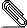# [tw] Re: requesting help with Template tiddlers

Hi,

I didn't like my last proposal. So here is a new one, that you can drag and
drop import to your TW.

The main code looks like this:

\define val(tid x)
<$transclude tiddler=$tid$index=$x$>$x$.</$transclude>
\end
\define registers(A:"." X:"." Y:"." N:"." V:"." Z:"." C:".")
Registers affected

| <<val $N$ N>> | <<val $V$ V>> | <<val $Z$ Z>> | <<val $C$ C>>|
| <<val $A$ A>> | <<val $X$ X>> | <<val $Y$ Y>> | |

\end

<<registers N:"*"  A:"*" Y:"*" C:"-">>

There are 2 loopup tiddlers in the json file.

* ... useful formats
- ... altered formats

The "." is default fallback in the val transclude macro.

If you have questions ... just ask

have fun!
mario

--
You received this message because you are subscribed to the Google Groups
"TiddlyWiki" group.
To unsubscribe from this group and stop receiving emails from it, send an emailregister-info-table.json